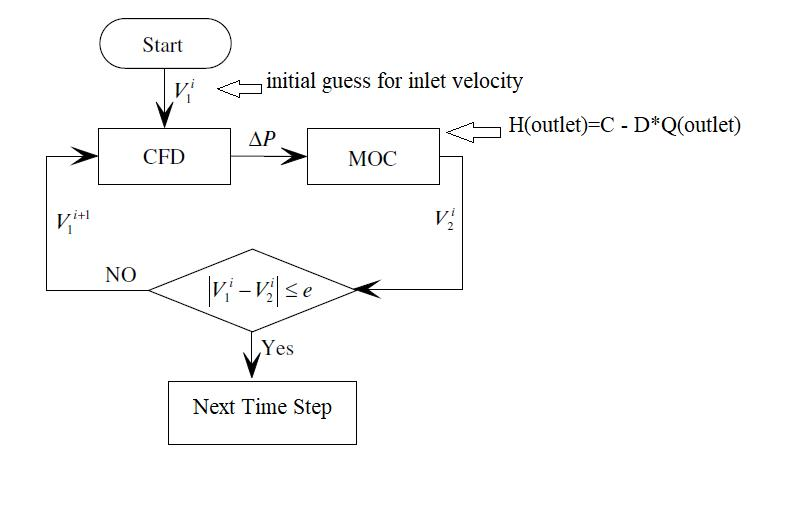## Fluids

Topics relate to Fluent, CFX, Turbogrid and more

•MohammadUT
Subscriber

Question: How can I make solver not to further in time after Max Iteration/Time step is done, and repeat the calculation with new B.C.s at same step of time util a criterion is met?
I have two simple equations which determine the pressure and velocity at the inlet and outlet of my CFD domain. Starting with an initial guess for these values, I want to couple these values to the result of Fluent and in each time step perform a loop till the values predicted by equations drop in a specific range of those predicted by Fluent.
So, basically I want to perform a loop without solver going to the next time step until a certain residual is reached.

•Rob
Ansys Employee

If you're altering the inlet & outlet conditions to suit an external model what are you solving in the Fluent case?

•MohammadUT
Subscriber

what I know from the B.C.s is the relation between Pressure and Flow rate as follow:

from Method of characteristics (external model):

Inlet:  H=known and Q=unknown;

outlet: H(outlet)=C(a known constant) - D(a known constant)*Q(outlet)

constants are obtained from values of H and Q at previous time step.

I want to couple these equations to fluent case and obtain the pressure drop = H(inlet)-H(outlet) as well as Q.

So basically the loop that I want to perform in each time step is going to find the H(outlet) and  Q.

•Rob
Ansys Employee

The CFD model geometry will give the relationship between pressure & flow. So you want to alter the outlet pressure and find the flow rate at that pressure? Given it's transient I have no idea how this will work (physically or computationally) as you're also working within a finite time with changing values.

Work through the logic and sketch out the physical process: that may make it easier to understand.

•MohammadUT
Subscriber

picture is from:

Wu, Dazhuan, et al. "MOC-CFD coupled approach for the analysis of the fluid dynamic interaction between water hammer and pump." Journal of Hydraulic Engineering 141.6 (2015): 06015003.•Rob
Ansys Employee

Thanks, I'm not sure you'll be able to do that within a time step. Run the system without the MOC loop and monitor pressure & flow to see what you get.

•MohammadUT
Subscriber

I know it can be done with Scheme programming (don't know how!), but is there any way to set flow time in UDF? Can I remain flow time constant till convergence of the above image is reached.

like:

(rpsetvar 'flow-time 0.2)

•Rob
Ansys Employee

You can call/push scheme from a UDF.